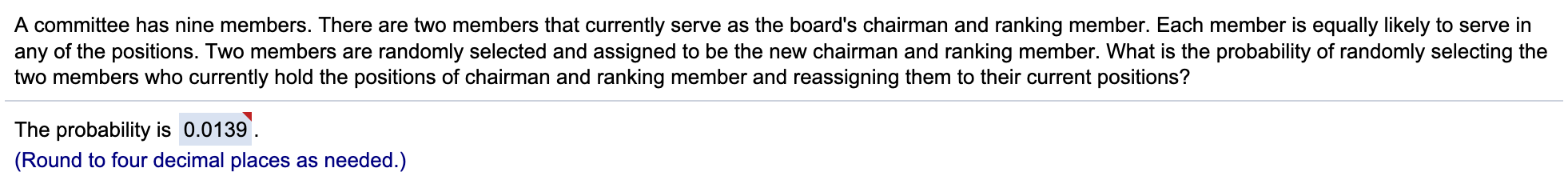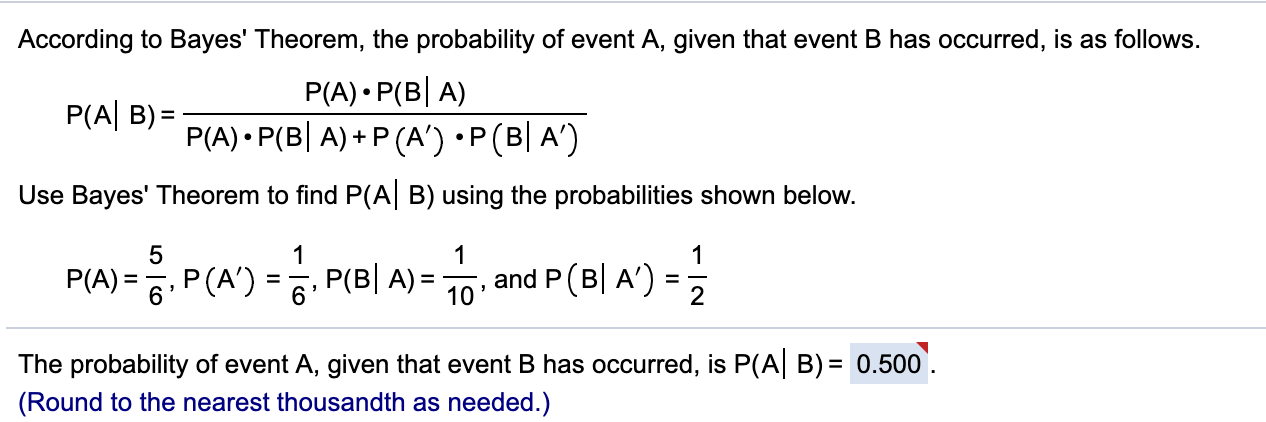# A committee has nine members. There are two members that currently serve as the board's chairman and ranking member. Each member is equally likely to serve inany of the positions. Two members are randomly selected and assigned to be the new chairman and ranking member. What is the probability of randomly selecting thetwo members who currently hold the positions of chairman and ranking member and reassigning them to their current positions?The probability is 0.0139.(Round to four decimal places as needed.) According to Bayes' Theorem, the probability of event A, given that event B has occurred, is as follows.P(A) P(B A)P(A) P(B A) P (A') P (B A')P(A B)=Use Bayes' Theorem to find P(A B) using the probabilities shown below.5111P(A)=6P(A) = P(B| A)= 10 , and P (B| A')-=2The probability of event A, given that event B has occurred, is P(A B) 0.500(Round to the nearest thousandth as needed.)

Question
347 views

I got the anwers for these questions on my quiz incorrect. Can you explain how to solve correctly?

For the first I attempted to solve with 2/9 possible chances to equal .2222

For the second I just imput the values given into the formula and recived the answer 1.083help_outlineImage TranscriptioncloseA committee has nine members. There are two members that currently serve as the board's chairman and ranking member. Each member is equally likely to serve in any of the positions. Two members are randomly selected and assigned to be the new chairman and ranking member. What is the probability of randomly selecting the two members who currently hold the positions of chairman and ranking member and reassigning them to their current positions? The probability is 0.0139. (Round to four decimal places as needed.) fullscreenhelp_outlineImage TranscriptioncloseAccording to Bayes' Theorem, the probability of event A, given that event B has occurred, is as follows. P(A) P(B A) P(A) P(B A) P (A') P (B A') P(A B)= Use Bayes' Theorem to find P(A B) using the probabilities shown below. 5 1 1 1 P(A)=6P(A) = P(B| A)= 10 , and P (B| A')- = 2 The probability of event A, given that event B has occurred, is P(A B) 0.500 (Round to the nearest thousandth as needed.) fullscreen
check_circle

star
star
star
star
star
1 Rating
Step 1

The probability of randomly selecting the two members who currently hold the positions of chairman and ranking member and reassigning them to their current positions is and it is obtained below:

From the given information, the committee has nine members. There are two members that currently serve as the board’...

### Want to see the full answer?

See Solution

#### Want to see this answer and more?

Solutions are written by subject experts who are available 24/7. Questions are typically answered within 1 hour.*

See Solution
*Response times may vary by subject and question.
Tagged in

### Other